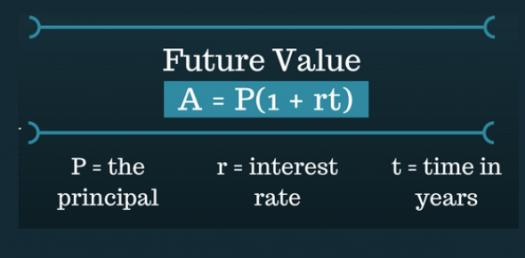# Basic Concepts Simple Interest

9 Questions | Total Attempts: 5900SettingsThis test involves the use of the simple interest formula to find, principal P, amount A, Rate R, Time in years, Simple InterestI let's begin and learn about it!

• 1.
Mr.   Smith deposited \$40, 000 in a bank and earned simple interest at 7 % per annum for two years. Calculate the interest earned at the end of the period.
• A.

\$ 4000

• B.

\$2800

• C.

\$5600

• D.

\$3400

• E.

\$200

• 2.
Principal + Interest = ,,,,,,,,,,,,,,,,,,,,,,,,,,,,,,,,,
• 3.
1. How much simple  interest  is due on a loan of  US\$120 for two years if the annual rate of interest is 5 ½ %.
• A.

\$12

• B.

\$13.20

• C.

\$26.40

• D.

\$26

• E.

\$33

• 4.
1. A man wishes to invest \$1500  . He can invest in   uLintplus Investment club which pays simple interest of 8%for 3 years. What is the amount in his account after 3 years
• A.

\$18321

• B.

\$1860

• C.

\$1830

• D.

\$1524

• E.

\$5024

• 5.
Calculate  the time T for \$1000 to become\$1300 at a rate of 5 %
• A.

3 years

• B.

6 years

• C.

9 years

• D.

6. 5 months

• E.

10 years

• 6.
Find the sum that amounts to \$2040 in 6 months at 4 %
• A.

\$ 200

• B.

\$2000

• C.

\$1300

• D.

\$2400

• E.

\$1020

• 7.
When you borrow money from the bank you pay
• 8.
.............................the percent charged or earned
• 9.
The amount of money borrowed or invested is called
Related TopicsBack to top